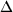### Raphaël Pélissier, Pierre Couteron, Stéphane Dray, and Daniel Sabatier. 2003. Consistency between ordination techniques and diversity measurements: two strategies for species occurrence data. Ecology 84:242–251.

Appendix C. Relationships between occurrence and contingency tables.

If Tc is a centred-by-columns occurrence table and X contains dummy variables corresponding to the modalities of a single qualitative variable, then between-analysis is the GSVD of (TcX, Dp, Dn), which can be expressed by introducing the projection operator onto X as:

TcXtDnTcXp = PX(Tc)tDnPX(Tc)p
= (X(XtDnX)-1XtDn Tc)tDnX(XtDnX)-1XtDnTcp
= TctDnX(XtDnX)-1 XtDnX(XtDnX)-1< b>XtDnTcp
= TctDnX(XtDnX)-1 XtDnTcp
= P0tDm-1P0p

where: P0 = XtDnTc = [fkj - fkfj] is the (m × p) centred table of the relative frequencies fkj of the occurrences that belong to modality k and species j; fk is the marginal frequency of modality k and fj the marginal frequency of species j; Dm-1 = (XtDnX)- 1 is (m × m) diagonal matrix containing modality weights equal to 1/fk.

The GSVD of (P0, Dp-1, Dm-1) is a Correspondence Analysis (CA; Hill 1974), while the GSVD of (P0, Ip, Dm-1) is a Non-Symmetric Correspondence Analysis (NSCA) on row profiles (Lauro and D'Ambra 1984).

Literature cited

Hill, M. O. 1974. Correspondence analysis: a neglegted multivariate method. Journal of the Royal Statistical Society 23:340–354.

Lauro, N., and L. D'Ambra 1984. L'analyse non symétrique des correspondances. Pages 433–446 in E. Diday, editor. Data analysis and informatics III, Elsevier, The Netherlands.

[Back to E084-006]